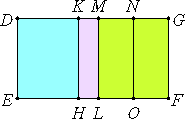# Proposition 62

The square on the second bimedial straight line applied to a rational straight line produces as breadth the third binomial.

Let AB be a second bimedial straight line divided into its medials at C, so that AC is the greater segment, let DE be any rational straight line, and to DE let there be applied the parallelogram DF equal to the square on AB and producing DG as its breadth.

I say that DG is a third binomial straight line.

Make the same construction as shown before.Then, since AB is a second bimedial divided at C, therefore AC and CB are medial straight lines commensurable in square only and containing a medial rectangle, so that the sum of the squares on AC and CB is also medial.

X.22

And it equals DL, therefore DL is also medial. And it is applied to the rational straight line DE, therefore MD is also rational and incommensurable in length with DE.

For the same reason, MG is also rational and incommensurable in length with ML, that is, with DE, therefore each of the straight lines DM and MG is rational and incommensurable in length with DE.

X.11

Since AC is incommensurable in length with CB, and AC is to CB as the square on AC is to the rectangle AC by CB, therefore the square on AC is also incommensurable with the rectangle AC by CB.

Hence the sum of the squares on AC and CB is incommensurable with twice the rectangle AC by CB, that is, DL is incommensurable with MF, so that DM is also incommensurable with MG.

X.36

And they are rational, therefore DG is binomial.

It is to be proved that it is a third binomial straight line.

In manner similar to the foregoing we may conclude that DM is greater than MG, and that DK is commensurable with KM.

And the rectangle DK by KM equals the square on MN, therefore the square on DM is greater than the square on MG by the square on a straight line commensurable with DM. And neither of the straight lines DM nor MG is commensurable in length with DE.

X.Def.II.3

Therefore DG is a third binomial straight line.

Therefore, the square on the second bimedial straight line applied to a rational straight line produces as breadth the third binomial.

Q.E.D.

## Guide

This proposition is used in X.72.